# 6th Grade Decimals Worksheets - Division

## Math worksheets: Division of decimals

Our decimal division worksheets are divided into sections: the first section provides questions in horizontal format; most of these can be done through mental math. The second section uses the long division format and emphasizes computation practice.

## Decimal Division

Decimals / whole numbers 1.84 ÷ 2 =
Decimals / whole numbers, (missing numbers) 0.8 ÷ ___ = 0.2

Decimals / decimals 6.2 ÷ 0.1 =
Decimals / decimals, (harder) 0.48 ÷ 0.06 =

Whole numbers / 10, 100, or 1,000 7,285 ÷ 100 =
Divide by 10/100/1,000, (missing numbers) 92 ÷ ____ = 0.92
Whole numbers / powers of 10 218,625 ÷ 1,000 =

Decimals / 10, 100, or 1000 2.83 ÷ 10 =
Decimals / powers of ten 3.375 ÷ 10,000 =

## Long division with decimals

Long division: decimals / whole numbers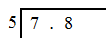Long division: decimals / whole numbers, (harder)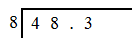Long division: decimals / whole numbers, (rounding)

Whole number division, (some repeating decimals)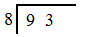Decimal long division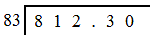Decimal / 1-digit decimal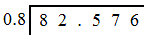Decimal / 2-digit decimal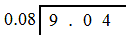Decimal / 3-digit decimal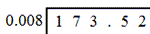Sample Grade 6 Decimal Division Worksheet

## More decimals worksheets

Find all of our decimals worksheets, from converting fractions to decimals to long division of multi-digit decimal numbers.

What is K5?

K5 Learning offers free worksheets, flashcards and inexpensive workbooks for kids in kindergarten to grade 5. Become a member to access additional content and skip ads.